### Home > MC2 > Chapter 2 > Lesson 2.3.6 > Problem2-153

2-153.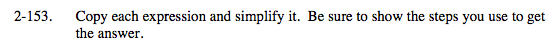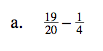Remember that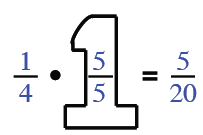$\frac{14}{20} = \frac{7}{10}$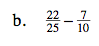One way to start is to change both so that they have 100 in the denominator.

$\frac{22}{25}\cdot \frac{4}{4} = \frac{88}{100}$

$\frac{7}{10}\cdot\frac{?}{?} = ?$

$\frac{9}{50}$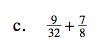See part (a).